Open in App
Not now

# Scheduling in Greedy Algorithms

• Difficulty Level : Easy
• Last Updated : 03 Nov, 2022

In this article, we will discuss various scheduling algorithms for Greedy Algorithms. Many scheduling problems can be solved using greedy algorithms.

Problem statement: Given N events with their starting and ending times, find a schedule that includes as many events as possible. It is not possible to select an event partially. Consider the below events: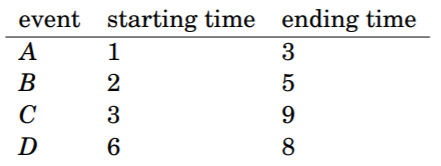• In this case, the maximum number of events is two. As selected events B and D are as follows: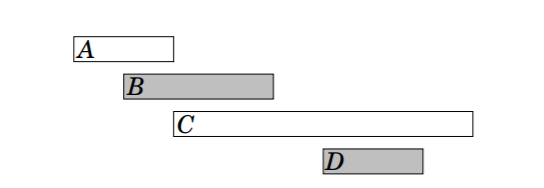• It is possible to invent several greedy algorithms for the problem.

Algorithms that work with every case:

Algorithm 1

• The first idea is to select as short events as possible. In the example, this algorithm selects the following events: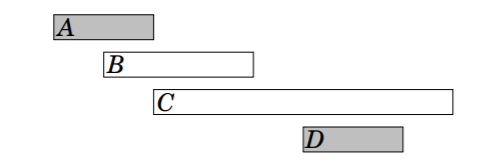• However, selecting short events is not always a correct strategy. For example, the algorithm fails in the below case:• If short event is selected, it can only select one event. However, it would be possible to select both long events.

Algorithm 2:

• Another idea is to always select the next possible event that begins as early as possible. This algorithm selects the following events: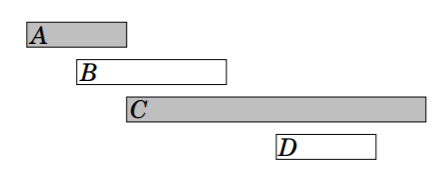• However, given a counter example for this algorithm. In this case, the algorithm only selects one event: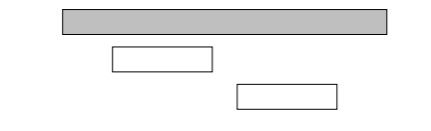• If the first event is selected, it is not possible to select any other events. However, it would be possible to select the other two events.

Algorithm 3:

• The third idea is to always select the next possible event that ends as early as possible. This algorithm selects the following events: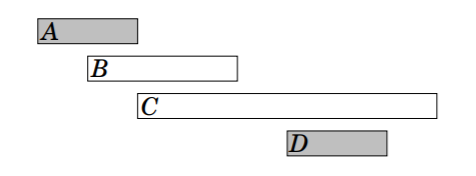• It turns out that this algorithm always produces an optimal solution.
• The reason for this is that it is always an optimal choice to first select an event that ends as early as possible.
• After this, it is an optimal choice to select the next event using the same strategy, etc., until any other event can’t be selected.
• One way the algorithm works is to consider what happens if first select an event that ends later than the event that ends as early as possible.
• Now, with having at most an equal number of choices how the next event can be selected.
• Hence, selecting an event that ends later can never yield a better solution, and the greedy algorithm is correct.
My Personal Notes arrow_drop_up